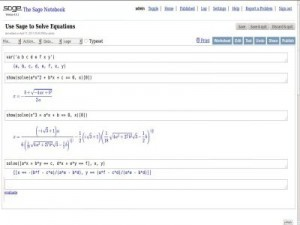# Top 20 MatLab Interview Questions & Answers

1) Explain what is MatLab? Where MatLab can be applicable?

MatLab is a high-level programming language with an interactive environment for visualization, numerical computation and programming function.

Matlab can be applicable at numerous instances like

• Allows matrix manipulations
• Plotting of functions and data
• Implementation of algorithms
• Creation of user interfaces
• Analyze data
• Develop algorithm
• Create models and applications
• Interfacing with programs written in other languages ( C++, C, Java and Fortran)

2) What does MatLab consist of?

MatLab consists of five main parts

• MatLab Language
• MatLab working environment
• Handle Graphics
• MatLab function library
• MatLab Application Program Interface (API)

3) Explain MatLab API (Application Program Interface)?

MatLab API is a library that enables you to write Fortran and C programs that interact with MatLab. It contains the facilities for calling routines from MatLab, for reading and writing Mat files and calling Matlab as a computational engine.

4) What are the types of loops does Matlab provides?

Matlab provides loops like

• While Loop
• For Loop
• Nested Loops

5) List out the operators that MatLab allows?

Matlab allows following Operators

• Arithmetic Operators
• Relational Operators
• Logical Operators
• Bitwise Operations
• Set OperationsSimulink is an add-on product to MatLab, it provides an interactive, simulating, graphical environment for modeling and analyzing of dynamic systems.

7) In MatLab is it possible to handle multi-dimensional arrays?

Yes, it is possible in MatLab to handle multi-dimensional arrays. Matlab’s internal data structure is limited to a two-dimensional matrix. But to handle multi-dimensional arrays in Matlab, you can create your own functions in Matlab language.

8) Mention what is the sign convention used in MatLab’s fft routines?

The sign convention used in MatLab’s fft routines are defined as sum(x(i)*exp (-j*i*k/N)) and not sum (x(i)exp(j*i*k/N)). The first version is used by engineers, and the second is used by mathematician.

9) What are the four basic functions to solve Ordinary Differential Equations (ODE)?

The four basic functions that MatLab has to solve ODE’s are

• ODE23
• ODE45

10) Explain how polynomials can be represented in MatLab?

A polynomial in MatLab is denoted by a vector. To create a polynomial in MatLab enter each co-efficient of the polynomial into the vector in descending order

11) What is the type of program files that MatLab allows to write?

Matlab allows two types of program files

• Scripts: It is a file with .m extension. In these files, it writes series of command that you want to execute together. It does not accept inputs and do not return any outputs

• Functions: They are also files with .m extension. Functions can accept inputs and return outputs.

12) Explain how to modify the MatLab Path?

To modify the MatLab Path use the PathTool GUI. Also, you can use add path directories from the command line and add the path to rc to write the current path back to ‘pathdef.m.’ In the case if you don’t have permission to write for ‘pathdef.m’ then pathrc can be written into a different file, you can execute from your ‘startup.m.’

13) Explain what is LaTex in MatLab?

MatLab handles naturally simple LaTex encoding which allows introducing greek letters or modifying the font size and appearance in plots.

14) Explain how you can pre-allocate a Non-Double Matrix?

Pre-allocating a block of memory for holding a non-double matrix is memory efficient. While allocating blocks of memory for a matrix, zeros are pre-allocated to a matrix.

The functions to pre allocate memory is int8(), example matrix =int8(zeros(100));

Repmat function is used to create a single double matrix, example matrix2=repmat(int8(0), 100, 100)

15) What is Xmath-Matlab? Mention the Xmath features?

For Xwindow workstations, Xmath is an interactive scripting and graphics environment.

Following are the X-math features

• Scripting language with OOP features
• Libraries that are LNX and C language compatible
• A debugging tools with GUI features
• Color graphics can be pointed and clickable

16) Name the graphic system used in MatLab?

Graphic system used in MatLab is known as handle graphics. It has a high level and low-level commands.

• High Level Commands: High level command performs image processing, data visualization and animation for 2D and 3D presentation graphics

• Low Level Commands: Full customization of the appearance of graphics and building of complete graphical user interface

17) Explain what is M-file and MEX files in MatLab?

M files: They are just a plain ASCII text that is interpreted at run time. They are like sub-programs stored in text files with .m extensions and are called M-files. For most of the MatLab, development M-files are used.

MEX files: They are basically native C or C++ files which are linked directly into the MatLab application at runtime. MEX files have efficiency to crash the MatLab application.

18) Explain what is Interpolation and Extrapolation in Matlab? What are their types?

• Interpolation: Taking out function values between different data points in an array is referred as Interpolation

• Extrapolation: Finding function values beyond the endpoints in array is referred as Extrapolation

The two types of Interpolation and Extrapolation are

• Linear Interpolation and Extrapolation

19) List out some of the common toolboxes present in Matlab?

Some of the common toolboxes in Matlab are

• Control System
• Fuzzy Logic
• Image Processing
• LMI control
• Neural Networks
• Robust Control
• System Identification

20) What is Get and Set in Matlab?

Get and Set are referred as getter and setter functions. For assigning properties, setter functions are used while for accessing properties getter functions are used.

6 replies

1.ali says:

How can a function block diagram in Matlab achieved?

2.ashwin kartheeban says:

You are wrong.
MULTI DIMENSIONAL ARRAY IS POSSIBLE AND BUILT IN AVAILABLE.

All the rest are ok.

•Guru99 says:

Thanks! it is now corrected

3.amanjot says:

Really full of knowledge.

4.5.